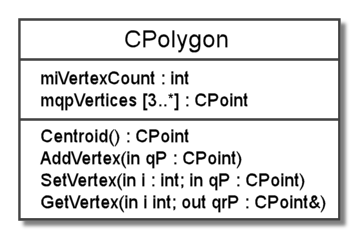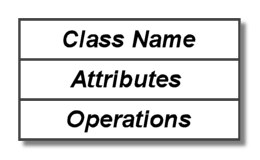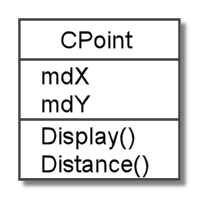# Bits & Bytes

## Archive for the ‘UML’ Category

### UML Class Diagrams – part 2

Continued . . .

Previously, we demonstrated a basic class diagram of a simple class that could be used for creating points in the plane. Now we will expand on the elements of what we showed before with some additional details in our UML class diagram. To be sure, we will still have more details to add when we are done here, but rest assured we will fill out everything in the end.

We begin with the C++ header code below for a polygon class, called CPolygon; this class could be used in conjunction with our previous point class to represent polygons in the plane. This polygon class consists of two member variables: an int to hold a count of the number of vertices in the polygon and a pointer to a dynamically allocated array of vertices that are CPoint objects. Additionally, there are four simple member functions to calculate the centroid (center point), add a vertex, set a vertex, and get a vertex. For simplicity, we have ignored constructors and the destructor.

```class CPolygon
{
public:
CPoint Centroid();
void SetVertex(int i, CPoint qP);
void GetVertex(int i, CPoint& qrP);

private:
int miVertexCount;
CPoint* mqpVertices;
};```

A UML diagram for this class is shown below. Notice that this diagram includes some details that we did not show previously. We have added the member variable types, which we tacked on with a colon after the variable names. Also, directly after the variable name mqpVertices, we have added [3..*] to represent the multiplicity of the vertices in our polygon (a polygon has three or more vertices). Presumbaly, the vertices array would be reallocated and copied when AddVertex() is called to add a vertex or something like that, but we have not specified the exact behavior here.For our operations, we add the return type after a colon. This is evident with the Centroid() function, since it has a return type of CPoint. The remaining functions have a void return type, so they return nothing. We could have shown a void return type, but we would probably never bother in actual practice. Likewise, we have omitted the void argument type for the Centroid() function. Unlike the return type, we can omit this, as we did, in the C++ code as well.

For the last three operations, we have member functions that take arguments. Since the AddVertex() function takes only one argument, we will start with it. Like our member variables, arguments have a name, then a colon, and finally the type of the argument. However, in AddVertex(), this is all preceded by the word “in” that is used to indicate that this is an input argument.

In this context, input means that the value that is passed in is important. In the GetVertex() function, the second argument is preceded by the word “out” to indicate that it is and output argument. In this case, the value that is passed in is not important, but the value that the argument variable has after the call is important. If both the input and output values are important, than we use “inout” to signify this. Lastly, notice that we replaced the commas that separated functional arguments in the C++ code with semicolons.

To be continued . . .

### UML Class Diagrams – part 1

UML stands for the Unified Modeling Language and it is a system for communicating the design and development of a software system. Object-oriented programming (OOP) has developed as the major tool to manage program complexity, and UML is the primary method of diagramming object-oriented programming structures and interactions. UML diagrams are used to create designs, communicate between developers, and document code so that it can be understood quickly. The most common type of UML diagram is a class diagram, which is sometimes called a static diagram.

A class diagram models classes and the relationships between them. The central element of class diagrams is a rectangular box that is used to represent a class. Typically, the box is separated into three sections that hold the class name, attributes, and operations. Attributes and operations are generic object-oriented design (OOD) terms for variables and functions. The layout of these items is shown here:A simple example class should help to make these abstractions concrete. The point class header shown below has two attributes that given by the x and y double variables that represent the coordinates of the point in the plane. The class also has two operations: one to display the point and one to find the distance to another point. The point class has four members in all, all of which are public.

```class CPoint
{
public:
double mdX;
double mdY;

void Display();
double Distance(CPoint qP);
};
```

With a basic illustration, this class can be represent by the simple UML diagram below. Notice that the member variables are in the attributes section and the member functions are in the operations section. That’s pretty straightforward, so far. However, this is just a simple diagram, and there are many other elements that we could have shown in it.Eventually, we will explain how to diagram this class completely and much more, but it is important to remember that diagrams do not need to display all of the details of the code. UML diagrams are for communication and understanding; they should be used to emphasize the elements that are important to the context. As we will demonstrate in our upcoming examples, extraneous details will often be omitted to highlight important points.

To be continued . . .